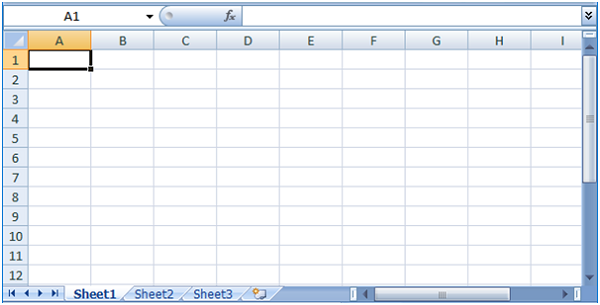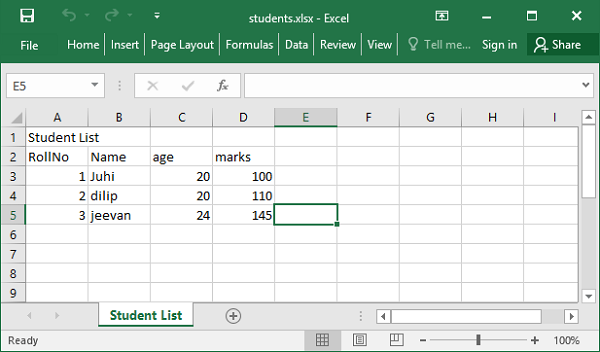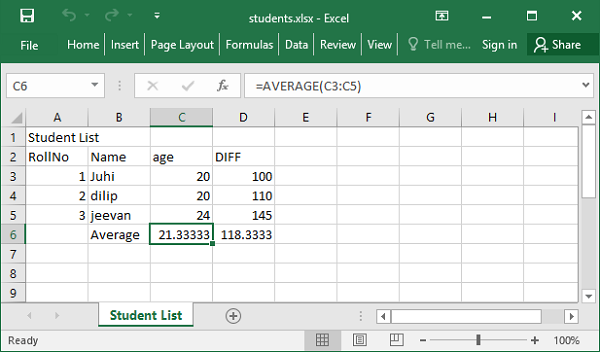# Data Persistence - Openpyxl Module

Microsoft’s Excel is the most popular spreadsheet application. It has been in use since last more than 25 years. Later versions of Excel use Office Open XML (OOXML) file format. Hence, it has been possible to access spreadsheet files through other programming environments.

OOXML is an ECMA standard file format. Python’s openpyxl package provides functionality to read/write Excel files with .xlsx extension.

The openpyxl package uses class nomenclature that is similar to Microsoft Excel terminology. An Excel document is called as workbook and is saved with .xlsx extension in the file system. A workbook may have multiple worksheets. A worksheet presents a large grid of cells, each one of them can store either value or formula. Rows and columns that form the grid are numbered. Columns are identified by alphabets, A, B, C, …., Z, AA, AB, and so on. Rows are numbered starting from 1.

A typical Excel worksheet appears as follows −The pip utility is good enough to install openpyxl package.

```pip install openpyxl
```

The Workbook class represents an empty workbook with one blank worksheet. We need to activate it so that some data can be added to the worksheet.

```from openpyxl import Workbook
wb=Workbook()
sheet1=wb.active
sheet1.title='StudentList'
```

As we know, a cell in worksheet is named as ColumnNameRownumber format. Accordingly, top left cell is A1. We assign a string to this cell as −

```sheet1['A1']= 'Student List'
```

Alternately, use worksheet’s cell() method which uses row and column number to identify a cell. Call value property to cell object to assign a value.

```cell1=sheet1.cell(row=1, column=1)
cell1.value='Student List'
```

After populating worksheet with data, the workbook is saved by calling save() method of workbook object.

```wb.save('Student.xlsx')
```

This workbook file is created in current working directory.

Following Python script writes a list of tuples into a workbook document. Each tuple stores roll number, age and marks of student.

```from openpyxl import Workbook
wb = Workbook()
sheet1 = wb.active
sheet1.title='Student List'
sheet1.cell(column=1, row=1).value='Student List'
studentlist=[('RollNo','Name', 'age', 'marks'),(1,'Juhi',20,100),
(2,'dilip',20, 110) , (3,'jeevan',24,145)]
for col in range(1,5):
for row in range(1,5):
sheet1.cell(column=col, row=1+row).value=studentlist[row-1][col-1]
wb.save('students.xlsx')
```

The workbook students.xlsx is saved in current working directory. If opened using Excel application, it appears as below −The openpyxl module offers load_workbook() function that helps in reading back data in the workbook document.

```from openpyxl import load_workbook
```

You can now access value of any cell specified by row and column number.

```cell1=sheet1.cell(row=1, column=1)
print (cell1.value)
Student List
```

### Example

Following code populates a list with work sheet data.

```from openpyxl import load_workbook
sheet1 = wb['Student List']
studentlist=[]
for row in range(1,5):
stud=[]
for col in range(1,5):
val=sheet1.cell(column=col, row=1+row).value
stud.append(val)
studentlist.append(tuple(stud))
print (studentlist)
```

### Output

```[('RollNo', 'Name', 'age', 'marks'), (1, 'Juhi', 20, 100), (2, 'dilip', 20, 110), (3, 'jeevan', 24, 145)]
```

One very important feature of Excel application is the formula. To assign formula to a cell, assign it to a string containing Excel’s formula syntax. Assign AVERAGE function to c6 cell having age.

```sheet1['C6']= 'AVERAGE(C3:C5)'
```

Openpyxl module has Translate_formula() function to copy the formula across a range. Following program defines AVERAGE function in C6 and copies it to C7 that calculates average of marks.

```from openpyxl import load_workbook